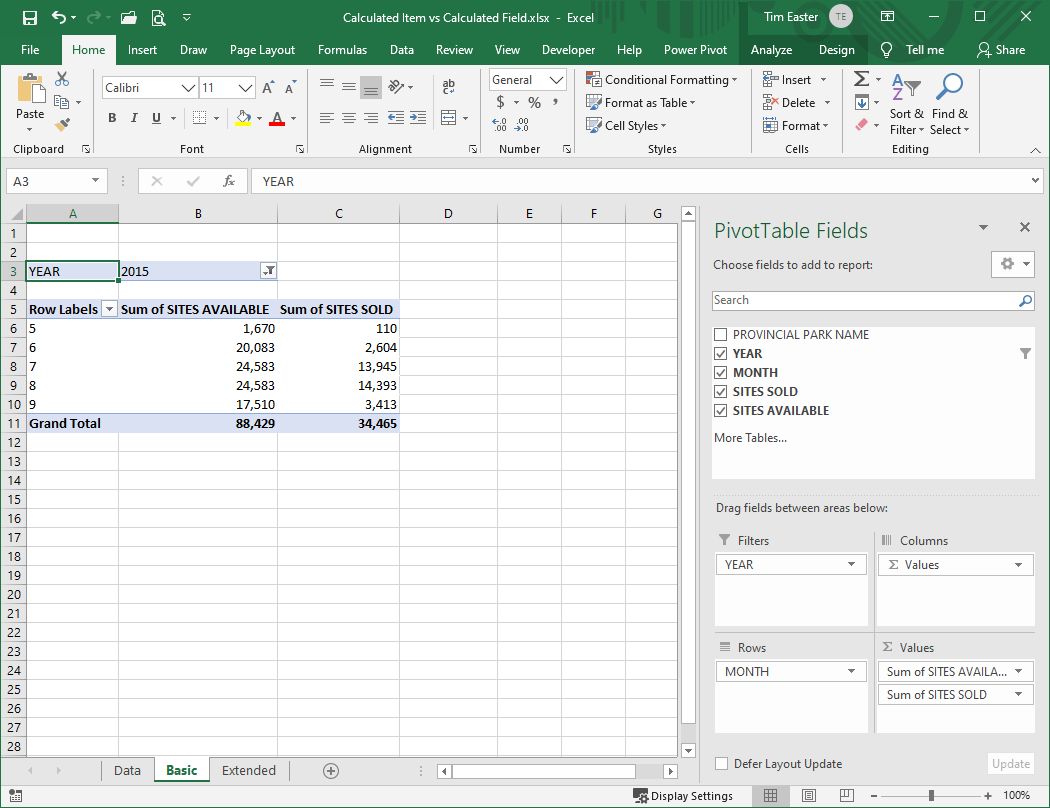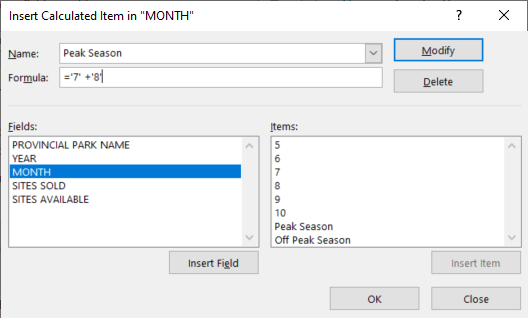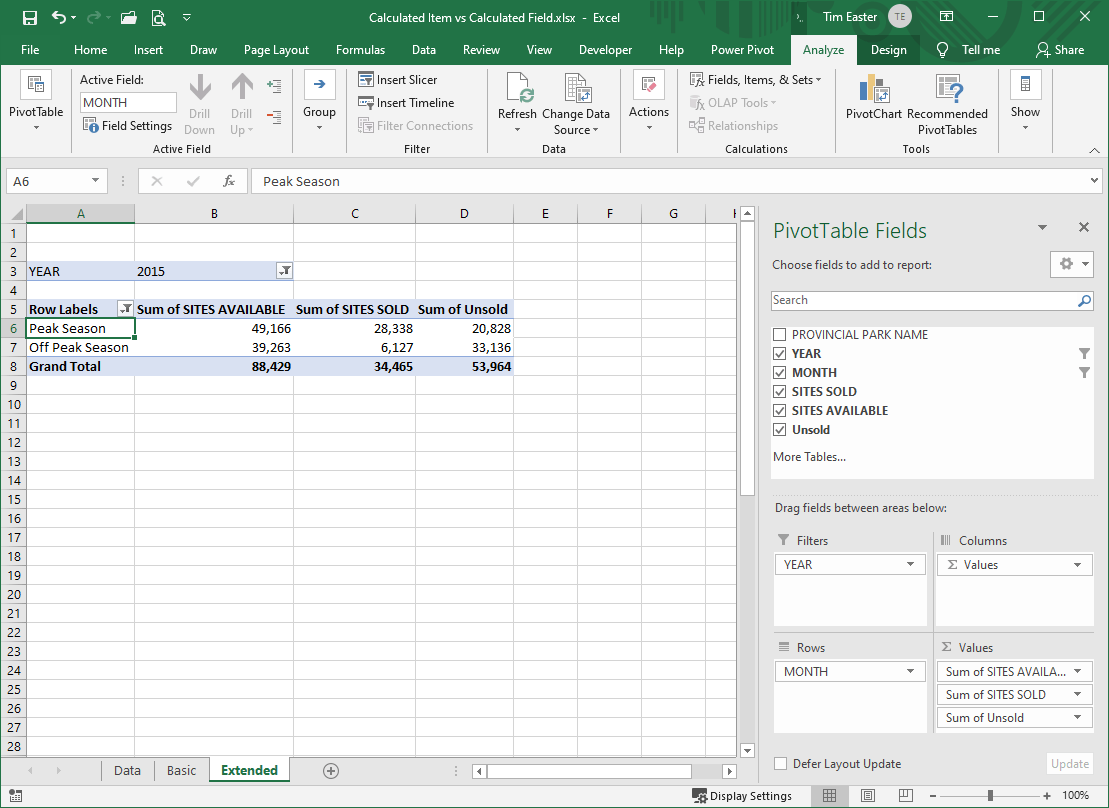## Calculated items vs. calculated fields

Pivot tables are powerful tools for summarizing and analyzing both small and large amounts of data.  Part of what makes them so powerful is the ability to create calculated items and calculated fields to extend what you can do with your base data (which becomes especially important when working on large data sets that you can’t easily modify).  If you are like me, though, the challenge is to remember the differences between them and what you use each one for.

Perhaps the key to keeping this straight is to remember what the words item and field mean in the context of a pivot table. If your source data is set up in a standard database layout (columns of different types of information and rows of data in each column), then think of items as the values in a row, and fields as the columns.  Or, items are the values that get summed up or otherwise analyzed, and fields are the types of information that you can place in filters, columns, or rows.  Thus, in the data below the value in cell C466 (or any other cell in the table) is an item, and column C is a field.When we summarize this data in a pivot table, we might have something like this, where the value 5 (for May) is an item in the field MONTH:With that in mind, here are some key features of items and fields, then we will look at how to use them.

Calculated Items

• Become an item in a field
• Can be used to sum other items in the same field or to perform other calculations
• Are listed with other values in the Row, or Column selection lists
• Do not appear in the list of Pivot Table Fields
• Cannot be placed in the filter area
• Individual records in the source data are calculated first, then the results are summed to create the item

Calculated Fields

• Become a field in the list of Pivot Table Fields and can be used like any other field
• Can perform calculations on other fields in the pivot table
• The value is determined based on the context it is placed in (i.e. the filters that are applied)
• Individual amounts referred to in the calculation are summed according to the context, then the calculation is performed on the totals

Let’s use an example on the data above to show how you might want to use each of these.

Calculated Item

First, while the data gives us information by month number, perhaps we want to summarize it by Peak Season (months 7 and 8) and Off Peak Season (all other months).  For this, we create two calculated items, Peak Season and Off Peak Season (go to Analyze, then Fields, Items & Sets, then Calculated Item).These will now show up in the pivot table as below:Note that the Grand Total is now double the original value, because each value appears both under the original month (e.g. month 5) and under the calculated item (e.g. Off Peak Season).  Therefore, we need to remember to deselect the values we don’t want to show to get back to the correct total.By doing this we have been able to view the data in a way that wasn’t possible from the original data.

But what if we want to know something from the data that requires a calculation between the various fields in the data?  For example, perhaps we want to look at the number of unsold sites.  Here is where a calculated field comes in.

Calculated Field

To do this, we go to Analyze, then Fields, Items, & Sets, then Calculated Field and create a field called Unsold, with the calculation = ‘SITES AVAILABLE’ – ‘SITES SOLD’, and add this to the pivot table values area, and now we can see the number of unsold sites.To summarize, using calculated items we can extend the values in the data set in various ways, and using calculated fields we can add new fields to open up further ways to analyze our data.  Using either or both, as in this example, we are able to move beyond the data we started with to find information based on calculations performed on the data in various ways. They are not without their limitations, but they are powerful features of pivot tables for certain circumstances.

Final note, if you are using the Data Model in Power Pivot to work with your data, you will need to use calculated measures and calculated columns, but that is a whole other subject for another day.